#### Optimal strategies

Now consider finding the optimal strategy, denoted by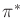, under the nondeterministic model. The sets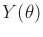for each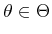must be used to determine which nature actions are possible for each observation,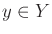. Let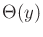denote this, which is obtained as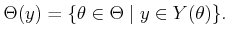(9.23)

The optimal strategy,, is defined by setting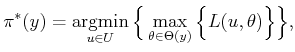(9.24)

for each. Compare this to (9.14), in which the maximum was taken over all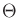. The advantage of having the observation,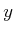, is that the set is restricted to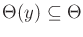.

Under the probabilistic model, an operation analogous to (9.23) must be performed. This involves computing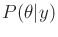from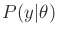to determine the information thatcontains regarding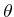. Using Bayes' rule, (9.9), with marginalization on the denominator, the result is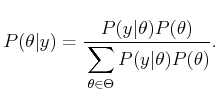(9.25)

To see the connection between the nondeterministic and probabilistic cases, define a probability distribution,, that is nonzero only if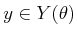and use a uniform distribution for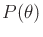. In this case, (9.25) assigns nonzero probability to precisely the elements ofas given in (9.23). Thus, (9.25) is just the probabilistic version of (9.23). The optimal strategy,, is specified for eachas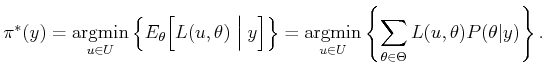(9.26)

This differs from (9.15) and (9.16) by replacingwith. For each, the expectation in (9.26) is called the conditional Bayes' risk. The optimal strategy,, always selects the strategy that minimizes this risk. Note thatin (9.26) can be expressed using (9.25), for which the denominator (9.26) represents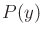and does not depend on; therefore, it does not affect the optimization. Due to this,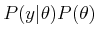can be used in the place ofin (9.26), and the samewill be obtained. If the spaces are continuous, then probability densities are used in the place of all probability distributions, and the method otherwise remains the same.

Steven M LaValle 2020-08-14